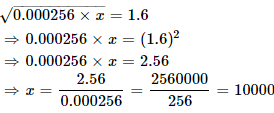Courses

# Test: Square Root And Cube Root- 1

## 10 Questions MCQ Test IBPS PO Mains - Study Material, Online Tests, Previous Year | Test: Square Root And Cube Root- 1

Description
This mock test of Test: Square Root And Cube Root- 1 for Quant helps you for every Quant entrance exam. This contains 10 Multiple Choice Questions for Quant Test: Square Root And Cube Root- 1 (mcq) to study with solutions a complete question bank. The solved questions answers in this Test: Square Root And Cube Root- 1 quiz give you a good mix of easy questions and tough questions. Quant students definitely take this Test: Square Root And Cube Root- 1 exercise for a better result in the exam. You can find other Test: Square Root And Cube Root- 1 extra questions, long questions & short questions for Quant on EduRev as well by searching above.
QUESTION: 1

###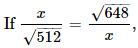find the value of x.

Solution: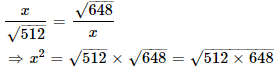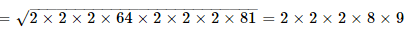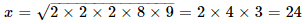QUESTION: 2

###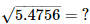Solution: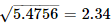QUESTION: 3

###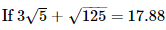then what will be the value of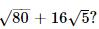Solution: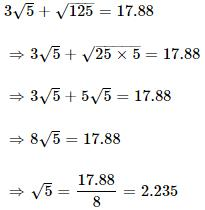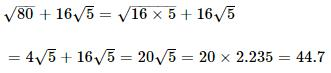QUESTION: 4

The cube root of 0.000729 is

Solution: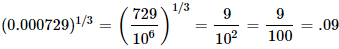QUESTION: 5

What is the least perfect square which is divisible by each of 21, 36 and 66?

Solution:

LCM of 21, 36, 66 = 2772

ie, all multiples of 2772 are divisible by 21, 36 and 66

Prime factorization of 2772 is,

2772 = 2 × 2 × 3 × 3 × 7 × 11

ie, to make it a perfect square, we have to multiply it by 7 and 11

Hence, required number = 2772 × 7 × 11 = 213444

QUESTION: 6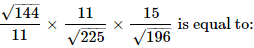Solution: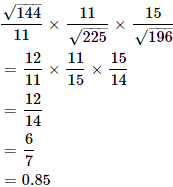QUESTION: 7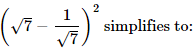Solution: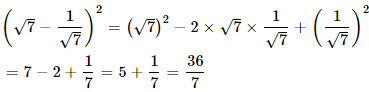QUESTION: 8

The square root of 16641 is

Solution: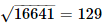QUESTION: 9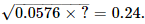Solution: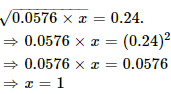QUESTION: 10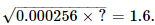Solution: